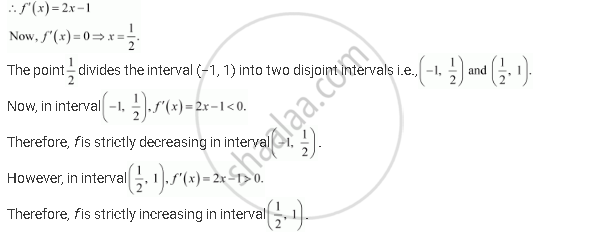Share

# Prove that the Function F Given By F(X) = X2 − X + 1 is Neither Strictly Increasing Nor Strictly Decreasing on (−1, 1). - CBSE (Commerce) Class 12 - Mathematics

ConceptIncreasing and Decreasing Functions

#### Question

Prove that the function f given by f(x) = x2 − x + 1 is neither strictly increasing nor strictly decreasing on (−1, 1).

#### Solution

The given function is f(x) = x2 − x + 1.Hence, f is neither strictly increasing nor decreasing in interval (−1, 1).

Is there an error in this question or solution?

#### APPEARS IN

NCERT Solution for Mathematics Textbook for Class 12 (2018 to Current)
Chapter 6: Application of Derivatives
Q: 11 | Page no. 206

#### Video TutorialsVIEW ALL 

Solution Prove that the Function F Given By F(X) = X2 − X + 1 is Neither Strictly Increasing Nor Strictly Decreasing on (−1, 1). Concept: Increasing and Decreasing Functions.
S